## Tuesday, 28 August 2012

### CLass 9 - Maths - Ch12 - HERON’S FORMULA

HERON’S FORMULA
(Questions from CBSE Papers)Points to Remember

1. Area of a triangle = ½ base × height
As in figure,
Area =  ½ BC × AM square units.2. When the triangle is right angled.

Area of a triangle = ½ base × height

= ½ × Product of sides containing the triangle.

3. When the triangle is equilateral i.e. all sides are of same length (say = a).

Take a mid-point on BC (say M) and join it to A.
Δ ABM is a right angles triangle.
Using Pythagoras theorem.

AB2 = AM2 + BM2

a2 = AM2 + (a/2)2
⇒ AM2 = a2 - a2/4 = 3a2/4
⇒  AM = (√3)a/2

Area of  Δ ABC = ½ × BC × AM = ½  × a × (√3)a/2
⇒   Area of  Δ ABC = (√3/4)a2

4. For an isosceles triangle, two sides are equal. As in figure in Δ ABC, AB = AC = a and BC = b.

Take a mid-point M on BC and draw a ⊥ AM from BC.
BM = MC = b/2
and AM = h

In rt-angled Δ ABM, using Pythagoras theorem:
AB2 = AM2 + BM2
⇒  AM2 =  AB2  - BM2
⇒  h2 = a2 - (b/2)2 = (4a2 - b2 )/4
Area of  Δ ABC = ½ × BC × AM = ½ × b × (4a2 - b2 )/4

4. For scalene triangles, since we do not know the height we cannot find area as we did in above cases.

5. Heron's Formula or Hero's Formula is the method to find area of any type of triangle, provided the length of the three sides are known. If a, b, c are the lengths of the triangle,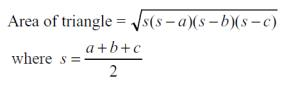s is the semi-perimeter of the triangle.

6. To compute the area of a quadrilateral whose sides and one diagonal are given. With the help of diagonal, partition the quadrilateral region into two triangular regions. Then apply Heron's formula to find the area of each triangle. The sum of  areas of the two triangles gives us the area of the quadrilateral.

Questions from CBSE Papers

Q1(CBSE 2010): The semi perimeter of a triangle having the length of its sides as 20 cm, 15 cm and 9 cm is:
(a) 44 cm
(b) 21 cm
(c) 22 cm
(d) none

Answer: (c) 22 cm

Q2(CBSE 2010/2011): Heron's formula is:

Q3: An isosceles triangle has perimeter 30 cm and each of the equal sides is 12 cm. Find area of the triangle.

Answer: An isosceles triangle has two sides equal a = b = 12 cm.
Given Perimeter = 30 cm
⇒ third side of the Δ is = c = 30 - (12 + 12) = 6 cm
Semi-perimeter s = 30 /2 = 15cm
Area of  Δ = [(15×(15-12)×(15-12)×(15-6)]1/2
⇒ = [15 × 3 × 3 × 9]1/2
⇒ = 9√(15) cm2

Q4: Find the area of an equilateral triangle with side 10 cm.

Answer:  Area of  equilateral Δ = (√3/4)a2  = (√3/4) × 102 = (√3/4) × 100 = 25√3 cm2
Using Heron's formula, s = 30/2 = 15
Area = [(15×(15-10)×(15-10)×(15-10)]1/2 = [15 × 5 × 5 × 5 ]1/2 = 25√3 cm2

Q5: A triangle and a parallelogram have the same base and the same area. If the sides of the triangle are 15 cm, 14 cm and 13 cm, and the parallelogram stands on the base 15 cm, find the height of the parallelogram.

Answer: Given, Area of Triangle = Area of the Parallelogram
and both have same base = 15cm.

Sides of the triangle are: a = 15cm, b = 14 cm and c = 13 cm
Semi-perimeter s = (15+14+13)/2 = 42/2 = 21 cm
Area of  Δ = [(21×(21-15)×(21-14)×(21-13)]1/2
= (21 × 6 × 7 × 8)1/2
= (3 × 7 × 2 × 3 × 7 × 2  × 4)1/2 = 3 × 7 × 2 × 2 = 84cm2
∴ Area of parallelogram = base  × height = 84
⇒ height = 84 / 15 = 5.6 cm

Q6: A traffic signal board, indicating ‘SCHOOL AHEAD’, is an equilateral triangle with side ‘a’. Find the area of the signal board, using Heron’s formula. If its perimeter is 180 cm, what will be the area of the signal board?

Answer: Each side of the equilateral  triangle = a
Semi-perimeter (s) = (a+a+a)/3 = 3a/2
Using Heron's formula, area of Δ = [(3a/2)×(3a/2 -a)×(3a/2 -a)×(3a/2 -a)]1/2
⇒ = [(3a/2)×(a/2)×(a/2)×(a/2)]1/2 =  a2/4(√3)
⇒ = a2√3/4
Given perimeter of  Δ = 180m = 3a
⇒ a = 60m
Therefore area of  Δ = (60)2√3/4 = 900√3 cm2

Q7: The sides of a Δ are 7 cm, 24 cm and 25 cm. Its area is :
(a) 168 cm2
(b) 84 cm2
(c) 87.5 cm2
(d) 300 cm2

Answer:  (b) 84 cm2
s = (7 + 24 + 25)/2 = 28
Area = [(28)×(28-7)×(28-24)×(28-25)]1/2  = [(28)×(21)×(4)×(3)]1/2
= [(7×4)×(7 ×3)×(4)×(3)]1/2  =  7 × 4 × 3 =  84 cm2

Q8: A square and an equilateral triangle have equal perimeters. If the diagonal of the square is 12√2cm, then area of the triangle is :
(a) 24√2 cm2
(b) 24√3 cm2
(c) 48√3 cm2
(d) 64√3 cm2

Answer:  (d) 64√3 cm2

Let side of square = a
Using Pythagoras theorem, 2a2 = 144 × 2 ⇒ a = 12cm
Perimeter of equilateral Δ = 4 × 12 = 48
Side of equilateral Δ (b) = 48/3 = 16cm
Area of equilateral Δ = (√3/4)b2 = (√3/4)(16)2 = 64√3 cm2

Miscellaneous

Q: How much area of a triangle will increase if its each side is doubled. Calculate the percentage increase.

Answer: Let a, b, c be the sides of the triangle.
Semi-perimeter (s1) = ½ (a + b + c)
The area of the Δ = A1 = [(s1)×(s1- a)×(s1- b)×(s1- a)]1/2
When the sides are doubled i.e. 2a, 2b and 2c,
Semi-perimeter (s2) = ½ (2a + 2b + 2c) = 2s1
The new area of  Δ = A2 = [(2s1)×(2s1- 2a)×(2s1- 2b)×(2s1- 2c)]1/2
⇒ A2 = [(2s1)×2(s1- a)×2(s1- b)×2(s1- c)]1/2  = 4 [(s1)×(s1- a)×(s1- b)×(s1- c)]1/2
⇒ A2 =  4A1
New area of Δ will be four times the area of original triangle.

% Increase = (A2- A1)/(A1) × 100 = (3A1)/(A1) × 100 = 300%

.1.More MCQ can be created

2.3.4.5.Thanks a lot for helping to complete my homework. This would be beneficial for exams.

6.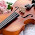this helps me a lot during my tests and exams.

7.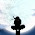good for exam preparations

8.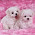9.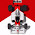Extra info was there

10.Extra info was there

11.This comment has been removed by the author.

12.Good link for students to learn who got stuck some times

13.Its nice website but it has very easy questions and answers of maths.....

14.Its nice website but it has very easy questions and answers of maths.....

15.This comment has been removed by the author.

16.Beneficial source to students who are not able to do self study of maths subject